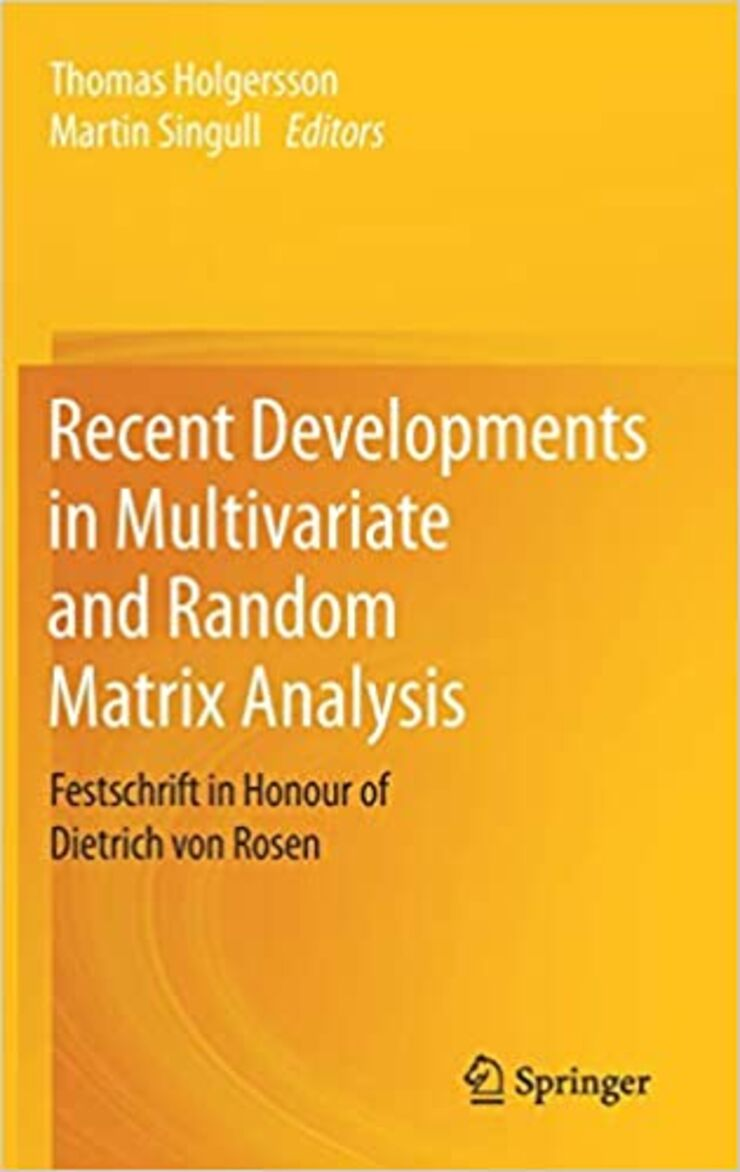# Martin Singull

## Multivariate Statistical Analysis

A covariance matrix is the simplest summary and the best way to explain the dependence relations of several variables. In different applications various structures for the covariance matrix could be of interest. The estimator for the covariance matrix is extra important since inference on the mean parameters strongly depends on the estimated covariance matrix. Hence, when testing the parameters for a multivariate model, in moderate and high dimensions, the estimator of the covariance matrix is very important.

For 200 years ago, Laplace and Gauss were some of the first ones to introduce the bivariate normal density, i.e., considering two dependent normal variables. In the end of 19th century Galton (half-cousin of Charles Darwin) exploited bivariate problems in genetics and introduced the ideas of correlation and regression in the study of paired measurements. Pearson developed the ideas further for studying problems in genetics, biology and other applications. In the beginning of 20th century Fisher gave the distribution of the sample Pearson correlation coefficient, where he also extended the chi-squared distribution to the bivariate case. This was later generalized by Wishart to the multivariate case and the Wishart matrix distribution which play an essential role in multivariate statistical analyses.

Many multivariate statistical analyses discussed in the literature are based on the assumption that the observation vectors are independent and normally distributed. The main reason for this is that sets of multivariate observations are often at least approximately normally distributed through the central limit effect. One advantage of normal distribution is that it is mathematically tractable and useful explicit results can be obtained. Furthermore, normally distributed data can also be modeled entirely in terms of their means and variances/covariances.

Estimating the mean and the covariance matrix is therefore a problem of great interest in statistics and it is also of course of great significance to consider the correct statistical model. Originally, many estimators of the covariance matrix were obtained from non-iterative least squares methods. When computer sources became stronger and covariance matrices with structures were considered iterative methods were introduced such as the maximum likelihood method and the restricted maximum likelihood method, among others.

Nowadays, more and more interests are in the area of high dimensional statistical analyses, i.e., when the dimension of the multivariate normal distribution is larger than the number of observations and even grows to infinity, and non-iterative methods have again become of interest. In multivariate analyses, especially in high dimensions, the behavior of eigenvalues and eigenvectors of random symmetric matrices are of much interest. These ideas goes back to the work of Pearson (early 20th century) who considered dimension reduction of multivariate data through principal component analysis. Furthermore, high dimensional statistical analyses and random matrices are very important tools in mathematics, financial mathematics, statistics, big data analyses, engineering, physics as well as other areas.

My research focuses on the problem of estimating and testing the parameters in multivariate normal distribution, in moderate and high dimensions, where the mean and covariance matrix may have various structures. For the covariance matrix it can be the Kronecker structure, which leads to a matrix normal distribution or more general the multilinear normal distribution, i.e., a tensor normal distribution. The Kronecker structured model can for example be used in the purpuse to model dependent multilevel observations. The mean can also follows a parametric model, such as linear, bilinear (also known as the Growth Curve model) or even higher dimensions as trilinear regression models. In these models the covariance matrix has a Kronecker product structure, whereas some factor matrices may be linearly structured, e.g., banded, intraclass, Toeplitz, circular Toeplitz, special structure with zeros or some mix.

## Professional activities

• Head of Division of Mathematical Statistics at the Department of Mathematics, Linköping University
• Assistant Director for the Research School in Interdisciplinary Mathematics at Linköping University
• Deputy Team Leader for the sub-programme Applied Mathematics and Statistics part of the University of Rwanda - Sweden Research, Higher Education and Institutional Advancement Cooperation Programme
• Member of the Board of the Department of Mathematics, Linköping University

## PhD studentsShow/Hide content

Former PhD students

## PublicationsShow/Hide content

### Books

Recent Developments in Multivariate and Random Matrix AnalysisRecent Developments in Multivariate and Random Matrix Analysis.Festschrift in Honour of Dietrich von Rosen
Edited by Thomas Holgersson and Martin Singull

This volume is a tribute to Professor Dietrich von Rosen on the occasion of his 65th birthday. It contains a collection of twenty original papers. The contents of the papers evolve around multivariate analysis and random matrices with topics such as high-dimensional analysis, goodness-of-fit measures, variable selection and information criteria, inference of covariance structures, the Wishart distribution and growth curve models.